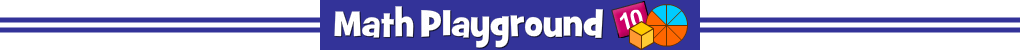Help the students of Trigon get to school on time.
Find the bus stop on the number line path.
Touch the number line to place each Trigonian.
Find the Bus Stop - Fractions and Decimals - Learning Connections
Essential Skills
Mental Math - practice locating fractions and decimals on a number line

Common Core Connection for Grades 3 and 4
Understand a fraction as a number on the number line.
Represent fractions on a number line diagram.
Understand two fractions as equivalent if they are the same point on a number line.
More Math Games to Play